Anzeige

# scrib.pptx

24. Mar 2023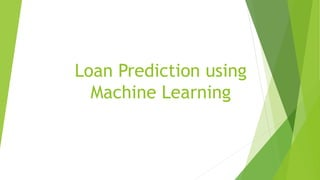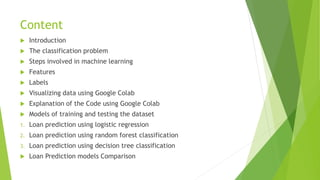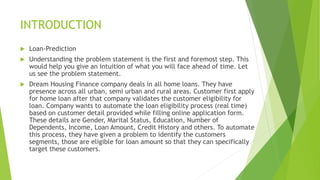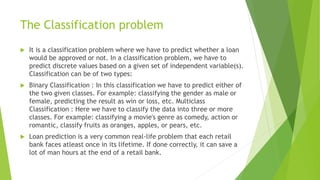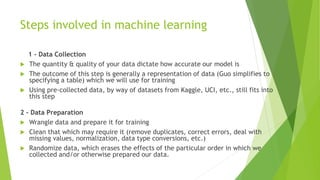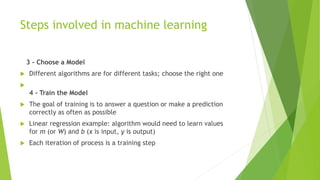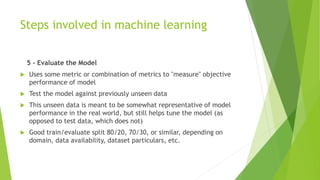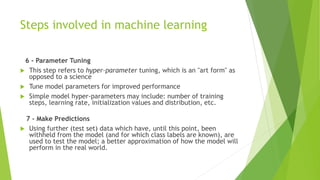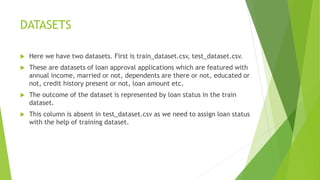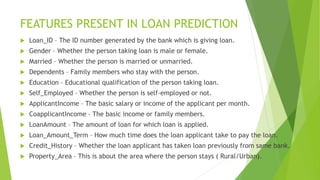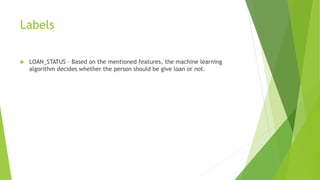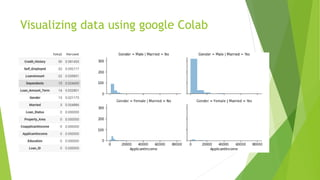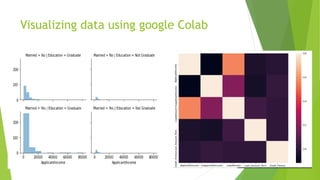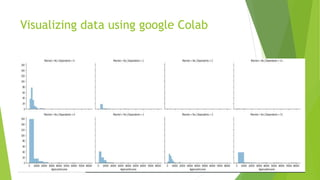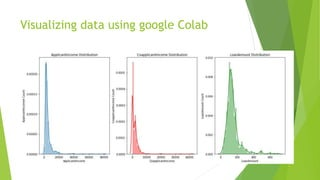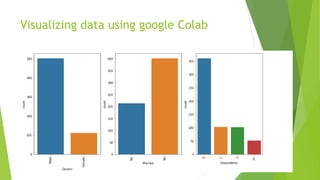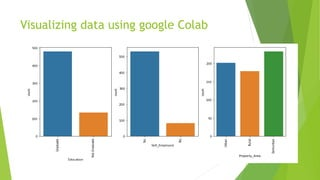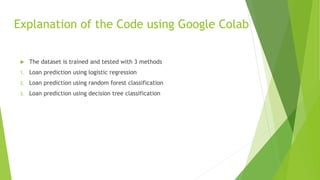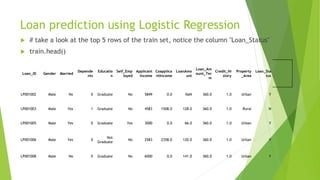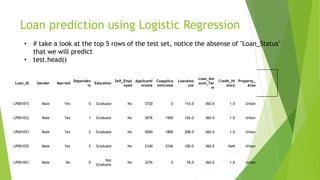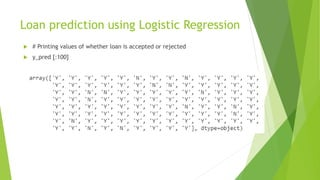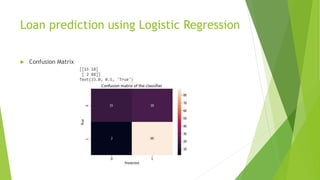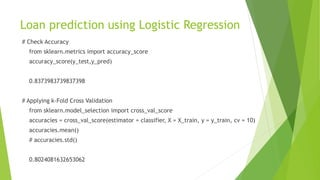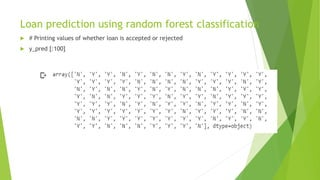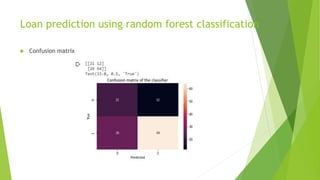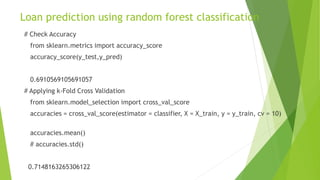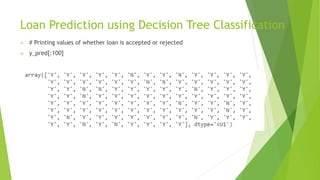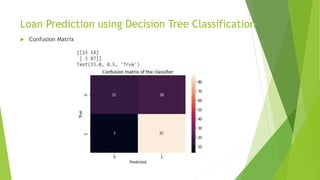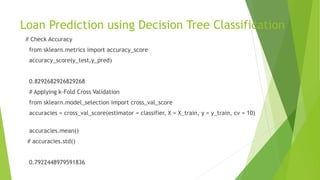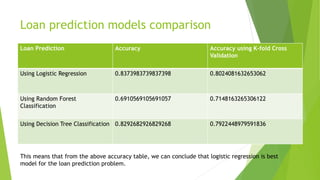1 von 31
Anzeige

### scrib.pptx

1. Loan Prediction using Machine Learning
2. Content  Introduction  The classification problem  Steps involved in machine learning  Features  Labels  Visualizing data using Google Colab  Explanation of the Code using Google Colab  Models of training and testing the dataset 1. Loan prediction using logistic regression 2. Loan prediction using random forest classification 3. Loan prediction using decision tree classification  Loan Prediction models Comparison
3. INTRODUCTION  Loan-Prediction  Understanding the problem statement is the first and foremost step. This would help you give an intuition of what you will face ahead of time. Let us see the problem statement.  Dream Housing Finance company deals in all home loans. They have presence across all urban, semi urban and rural areas. Customer first apply for home loan after that company validates the customer eligibility for loan. Company wants to automate the loan eligibility process (real time) based on customer detail provided while filling online application form. These details are Gender, Marital Status, Education, Number of Dependents, Income, Loan Amount, Credit History and others. To automate this process, they have given a problem to identify the customers segments, those are eligible for loan amount so that they can specifically target these customers.
4. The Classification problem  It is a classification problem where we have to predict whether a loan would be approved or not. In a classification problem, we have to predict discrete values based on a given set of independent variable(s). Classification can be of two types:  Binary Classification : In this classification we have to predict either of the two given classes. For example: classifying the gender as male or female, predicting the result as win or loss, etc. Multiclass Classification : Here we have to classify the data into three or more classes. For example: classifying a movie's genre as comedy, action or romantic, classify fruits as oranges, apples, or pears, etc.  Loan prediction is a very common real-life problem that each retail bank faces atleast once in its lifetime. If done correctly, it can save a lot of man hours at the end of a retail bank.
5. Steps involved in machine learning 1 - Data Collection  The quantity & quality of your data dictate how accurate our model is  The outcome of this step is generally a representation of data (Guo simplifies to specifying a table) which we will use for training  Using pre-collected data, by way of datasets from Kaggle, UCI, etc., still fits into this step 2 - Data Preparation  Wrangle data and prepare it for training  Clean that which may require it (remove duplicates, correct errors, deal with missing values, normalization, data type conversions, etc.)  Randomize data, which erases the effects of the particular order in which we collected and/or otherwise prepared our data.
6. Steps involved in machine learning 3 - Choose a Model  Different algorithms are for different tasks; choose the right one  4 - Train the Model  The goal of training is to answer a question or make a prediction correctly as often as possible  Linear regression example: algorithm would need to learn values for m (or W) and b (x is input, y is output)  Each iteration of process is a training step
7. Steps involved in machine learning 5 - Evaluate the Model  Uses some metric or combination of metrics to "measure" objective performance of model  Test the model against previously unseen data  This unseen data is meant to be somewhat representative of model performance in the real world, but still helps tune the model (as opposed to test data, which does not)  Good train/evaluate split 80/20, 70/30, or similar, depending on domain, data availability, dataset particulars, etc.
8. Steps involved in machine learning 6 - Parameter Tuning  This step refers to hyper-parameter tuning, which is an "art form" as opposed to a science  Tune model parameters for improved performance  Simple model hyper-parameters may include: number of training steps, learning rate, initialization values and distribution, etc. 7 - Make Predictions  Using further (test set) data which have, until this point, been withheld from the model (and for which class labels are known), are used to test the model; a better approximation of how the model will perform in the real world.
9. DATASETS  Here we have two datasets. First is train_dataset.csv, test_dataset.csv.  These are datasets of loan approval applications which are featured with annual income, married or not, dependents are there or not, educated or not, credit history present or not, loan amount etc.  The outcome of the dataset is represented by loan status in the train dataset.  This column is absent in test_dataset.csv as we need to assign loan status with the help of training dataset.
10. FEATURES PRESENT IN LOAN PREDICTION  Loan_ID – The ID number generated by the bank which is giving loan.  Gender – Whether the person taking loan is male or female.  Married – Whether the person is married or unmarried.  Dependents – Family members who stay with the person.  Education – Educational qualification of the person taking loan.  Self_Employed – Whether the person is self-employed or not.  ApplicantIncome – The basic salary or income of the applicant per month.  CoapplicantIncome – The basic income or family members.  LoanAmount – The amount of loan for which loan is applied.  Loan_Amount_Term – How much time does the loan applicant take to pay the loan.  Credit_History – Whether the loan applicant has taken loan previously from same bank.  Property_Area – This is about the area where the person stays ( Rural/Urban).
11. Labels  LOAN_STATUS – Based on the mentioned features, the machine learning algorithm decides whether the person should be give loan or not.
12. Visualizing data using google Colab
13. Visualizing data using google Colab
14. Visualizing data using google Colab
15. Visualizing data using google Colab
16. Visualizing data using google Colab
17. Visualizing data using google Colab
18. Explanation of the Code using Google Colab  The dataset is trained and tested with 3 methods 1. Loan prediction using logistic regression 2. Loan prediction using random forest classification 3. Loan prediction using decision tree classification
19. Loan prediction using Logistic Regression  # take a look at the top 5 rows of the train set, notice the column "Loan_Status"  train.head() Loan_ID Gender Married Depende nts Educatio n Self_Emp loyed Applicant Income Coapplica ntIncome LoanAmo unt Loan_Am ount_Ter m Credit_Hi story Property _Area Loan_Sta tus LP001002 Male No 0 Graduate No 5849 0.0 NaN 360.0 1.0 Urban Y LP001003 Male Yes 1 Graduate No 4583 1508.0 128.0 360.0 1.0 Rural N LP001005 Male Yes 0 Graduate Yes 3000 0.0 66.0 360.0 1.0 Urban Y LP001006 Male Yes 0 Not Graduate No 2583 2358.0 120.0 360.0 1.0 Urban Y LP001008 Male No 0 Graduate No 6000 0.0 141.0 360.0 1.0 Urban Y
20. Loan prediction using Logistic Regression Loan_ID Gender Married Dependen ts Education Self_Empl oyed ApplicantI ncome Coapplica ntIncome LoanAmo unt Loan_Am ount_Ter m Credit_Hi story Property_ Area LP001015 Male Yes 0 Graduate No 5720 0 110.0 360.0 1.0 Urban LP001022 Male Yes 1 Graduate No 3076 1500 126.0 360.0 1.0 Urban LP001031 Male Yes 2 Graduate No 5000 1800 208.0 360.0 1.0 Urban LP001035 Male Yes 2 Graduate No 2340 2546 100.0 360.0 NaN Urban LP001051 Male No 0 Not Graduate No 3276 0 78.0 360.0 1.0 Urban • # take a look at the top 5 rows of the test set, notice the absense of "Loan_Status" that we will predict • test.head()
21. Loan prediction using Logistic Regression  # Printing values of whether loan is accepted or rejected  y_pred [:100]
22. Loan prediction using Logistic Regression  Confusion Matrix
23. Loan prediction using Logistic Regression # Check Accuracy from sklearn.metrics import accuracy_score accuracy_score(y_test,y_pred) 0.8373983739837398 # Applying k-Fold Cross Validation from sklearn.model_selection import cross_val_score accuracies = cross_val_score(estimator = classifier, X = X_train, y = y_train, cv = 10) accuracies.mean() # accuracies.std() 0.8024081632653062
24. Loan prediction using random forest classification  # Printing values of whether loan is accepted or rejected  y_pred [:100]
25. Loan prediction using random forest classification  Confusion matrix
26. Loan prediction using random forest classification # Check Accuracy from sklearn.metrics import accuracy_score accuracy_score(y_test,y_pred) 0.6910569105691057 # Applying k-Fold Cross Validation from sklearn.model_selection import cross_val_score accuracies = cross_val_score(estimator = classifier, X = X_train, y = y_train, cv = 10) accuracies.mean() # accuracies.std() 0.7148163265306122
27. Loan Prediction using Decision Tree Classification  # Printing values of whether loan is accepted or rejected  y_pred[:100]
28. Loan Prediction using Decision Tree Classification  Confusion Matrix
29. Loan Prediction using Decision Tree Classification # Check Accuracy from sklearn.metrics import accuracy_score accuracy_score(y_test,y_pred) 0.8292682926829268 # Applying k-Fold Cross Validation from sklearn.model_selection import cross_val_score accuracies = cross_val_score(estimator = classifier, X = X_train, y = y_train, cv = 10) accuracies.mean() # accuracies.std() 0.7922448979591836
30. Loan prediction models comparison Loan Prediction Accuracy Accuracy using K-fold Cross Validation Using Logistic Regression 0.8373983739837398 0.8024081632653062 Using Random Forest Classification 0.6910569105691057 0.7148163265306122 Using Decision Tree Classification 0.8292682926829268 0.7922448979591836 This means that from the above accuracy table, we can conclude that logistic regression is best model for the loan prediction problem.
31. THANK YOU
Anzeige